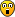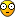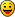## Easter egg graphics

Discussions about the Liberty BASIC language, with particular reference to LB Booster
tsh73
Posts: 36
Joined: Fri Apr 06, 2018 7:58 pm

### Easter egg graphics

Old program of mine a bit cleaned up
Works in lb/lbb
(would work in JB if supply custom sort routine - version on JB board)easterEgg.PNG (95.41 KiB) Viewed 660 times

Code: Select all

``````'3d easter egg (beaded)
'by tsh73, January 2009
'revised April 2018 - text added
'lb/lbb: qsort changed to native sort

nomainwin

DIM p(10000, 6)
ltrW=22
dim ltr\$(ltrW) 'Times New Roman italic via GetPixelValue\$
'hand-tuned a little bit
ltr\$( 1)="                               "
ltr\$( 2)="      **   ***                 "
ltr\$( 3)="    ***** ****                 "
ltr\$( 4)="      *** *  *                 "
ltr\$( 5)="       ** *                    "
ltr\$( 6)="       ****                    "
ltr\$( 7)="        **                     "
ltr\$( 8)="        **                     "
ltr\$( 9)="        **         *********   "
ltr\$(10)="       * **         ****  ***  "
ltr\$(11)="      *  **         ***   ***  "
ltr\$(12)="      *  **  *      ***   ***  "
ltr\$(13)=" **  *   **  *      ***  ***   "
ltr\$(14)=" ****     ****     ***  **     "
ltr\$(15)=" ***      **       ******      "
ltr\$(16)="                   ***  ***    "
ltr\$(17)="                   ***   ***   "
ltr\$(18)="                  ***    ***   "
ltr\$(19)="                  ***    ***   "
ltr\$(20)="                  ***   ****   "
ltr\$(21)="                 ***   ****    "
ltr\$(22)="                *********      "
ltrH=len(ltr\$(1))

global width, minX, maxX, height, minY, maxY, sqr3half, Pi
'some constants
sqr3half = SQR(3) / 2
Pi = acs(-1)

'indeed for 60 bands pearls look real size. That is, too small
'So I scale it up twice
WindowWidth = 600
WindowHeight = 600+25

UpperLeftX=int((DisplayWidth-WindowWidth)/2)
UpperLeftY=int((DisplayHeight-WindowHeight)/2)

open "Beaded Easter Egg" for graphics_nsb_nf as #grFunc
#grFunc, "trapclose [trapclosehLabel]"
#grFunc, "home ; down ; posxy x y"
'x, y give us width, height
width = 2*x : height = 2*y
#grFunc, "fill darkgreen; color white; flush"

minX = -1.5
maxX = 1.5
minY = -1.5
maxY = 1.5

nBands = 60
nMaxCols = 86

'experimentally I got 40 beads of r=10 for circle r = 1
'that is, for 2Pi, or 40/2/Pi per length unit.
n = nBands
i = 1

for k = 1 to n-1    'parallels (bands)
phi = Pi*k/n - Pi/2
rParr = cos(phi)    'this is band radius
'change sphere to egg
d = 1/6    'slant
kk = 0.75    'how narrow
rParr =  rParr * kk*((1-sin(phi))*d+(1-d))
nn = int(nMaxCols*rParr/kk)

'parallel at phi
alpha0=Pi*.65 'rotation, where j=0 is
FOR j = 0 TO nn-1
SCAN
'alpha = 2*Pi*i/nn'+Pi/2
alpha = alpha0-2*Pi*j/nn

x = cos(alpha)*rParr
y = sin(alpha)*rParr
z = sin(phi)

p(i, 1) = x
p(i, 2) = y
p(i, 3) = z    'radial bands
'p(i, 3) = z+(alpha-alpha0-Pi)/5    'spiral. doesn't exactly work
p(i, 4) = fnx(x, y, z)
p(i, 5) = fny(x, y, z)
'PSET (p(i, 4), p(i, 5)) 'draw
xx =  sx(p(i, 4))
yy =  sy(p(i, 5))
p(i, 6) = 0
'read bitmap from ltr\$() array.
offY=k-24
offX=j
if offY <= ltrW and offY >0 _
and offX < ltrH and offX>0 then
if mid\$(ltr\$(ltrW-offY+1),offX,1)="*" then p(i, 6)=1    'It reads upside down
end if
'uncomment to see 'zero' position
'if k = 30  then p(i, 6)=1    'about middle line
'if j=0 then p(i, 6)=1
i = i+1
NEXT
next
n = i-1

'now, paint them in Z-order
dim ord(n,2)
for i = 1 to n
ord(i,1) = i
ord(i,2) = p(i, 1)+p(i, 2)+p(i, 3)    'this works as Zorder for izometry
next
print "Sorting..."
t0 = time\$("ms")
sort ord(),1, n, 2
print "Sorting done, took "; time\$("ms")- t0
'then redraw in order.
for i = 1 to n
SCAN    'so you can break it
xx =  sx(p(ord(i,1), 4))
yy =  sy(p(ord(i,1), 5))
colNum = p(ord(i,1), 3)  ' = z
if p(ord(i,1), 6)=1 then colNum = 1
if n mod 100 = 0 then  #grFunc, "discard"
next

#grFunc, "flush"
wait

end

[trapclosehLabel]
close #grFunc
end

'***********************************************************************
[HSV_2_RGB]
'Input: (h,s,v)
'h in the range [0, 360), indicating the angle, in degrees of the hue
's and v varying between 0 and 1, representing the saturation and value, respectively
'Output: r,g,b  [0,1]
'and to be useful, R G B [0 255]
'or to JB RGB\$ as "R G B" string.

hi = int(h/60) mod 6
f = h/60 - int(h/60)
p = v*(1-s)
q = v*(1-f*s)
t = v*(1-(1-f)*s)
'    print hi,
select case hi
case 0
r = v: g = t: b = p
case 1
r = q: g = v: b = p
case 2
r = p: g = v: b = t
case 3
r = p: g = q: b = v
case 4
r = t: g = p: b = v
case 5
r = v: g = p: b = q
end select
R = int(r*255)
G = int(g*255)
B = int(b*255)
RGB\$= R;" ";G;" ";B
return

'***********************************************************************
h = (colNum+1)*180 'continuous band color
'h = (colNum+1)*1000 'continuous band color variant that looks pretty random
s=1
v=.5

for currR = beadR to 1 step -1
gosub [HSV_2_RGB]
#grFunc, "color ";RGB\$;";backcolor ";RGB\$
#grFunc, "circlefilled ";currR
next
return

'***********************************************************************
'3d to plabe
function fnx(x, y, z)
fnx = (x - y) * sqr3half
end function

function fny(x, y, z)
fny = z - (x + y) / 2
end function

'conversions (logical coords to screen)
function sx(x)
'screen X. Depends on width, minX, maxX
sx = (x- minX)/(maxX-minX) * width
end function

function sy(y)
'screen Y. Depends on height, minY, maxY. Upside down.
sy = (1-(y- minY)/(maxY-minY)) * height
end function
``````

guest
Posts: 64
Joined: Tue Apr 03, 2018 1:34 pm

### Re: Easter egg graphics

tsh73 wrote:
Wed Apr 11, 2018 8:53 pm
Old program of mine a bit cleaned up
Very nice!

Richard.

sarmednafi
Posts: 21
Joined: Fri Apr 06, 2018 6:27 am

### Re: Easter egg graphics

Very Very Very Nice...!This guy is Talent.